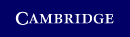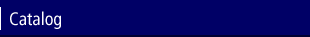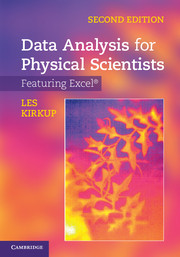Home > Catalog > Data Analysis for Physical Scientists## Details

• 149 b/w illus. 214 exercises
• Page extent: 528 pages
• Size: 247 x 174 mm
• Weight: 1.18 kg

## Library of Congress

• Dewey number: 502.85/554
• Dewey version: 23
• LC Classification: Q180.55.S7 K57 2011
• LC Subject headings:
• Research--Statistical methods--Data processing
• Electronic spreadsheets
• Microsoft Excel (Computer file)
• SCIENCE / Mathematical Physics.--bisacsh

Library of Congress Record

### Hardback

(ISBN-13: 9780521883726)

#### In stock

##### \$84.99 (P)

The ability to summarise data, compare models and apply computer-based analysis tools are vital skills necessary for studying and working in the physical sciences. This textbook supports undergraduate students as they develop and enhance these skills. Introducing data analysis techniques, this textbook pays particular attention to the internationally recognised guidelines for calculating and expressing measurement uncertainty. This new edition has been revised to incorporate Excel® 2010. It also provides a practical approach to fitting models to data using non-linear least squares, a powerful technique which can be applied to many types of model. Worked examples using actual experimental data help students understand how the calculations apply to real situations. Over 200 in-text exercises and end-of-chapter problems give students the opportunity to use the techniques themselves and gain confidence in applying them. Answers to the exercises and problems are given at the end of the book.

### Contents

1. Introduction to scientific data analysis; 2. Excel and data analysis; 3. Data distributions I; 4. Data distributions II; 5. Measurement, error and uncertainty; 6. Least squares I; 7. Least squares II; 8. Non-linear least squares; 9. Tests of significance; 10. Data analysis tools in Excel and the Analysis ToolPak; Appendixes; Answers to exercises and end-of-chapter problems; References; Index.

### Reviews

Reviews of the first edition: ‘This book is extremely well structured. It both describes the main functionality of Excel with special emphasis on scientific data analysis, as well as the statistical background to the methods … definitely one of the best on the market in this important area … the author should be congratulated on doing a wonderful job.' Richard Brereton, Chemistry Industry

‘Overall, I found the book excellent.' S. Middleton, The Physicist

Review of previous edition: ‘… a simple and straightforward introduction to the use of spreadsheet calculations and data display … The coverage of this book will be more than adequate for undergraduate courses and will be sufficient for many postgraduate and research readers … a good introduction to Excel® for data analysis for the first time user and covers the data analysis methods that most physical scientists will need.' B. W. James, Contemporary Physics

'The fundamental observation of experimental science is that repeated measurements of the same quantity by the same person using the same equipment do not repeatedly give the same value. … Thus, statistical analysis of experimental data is a large part of experimental physics … [This book] deals with all these problems at a level appropriate to an undergraduate course on data analysis. … introduces useful ideas and many examples on data visualisation, with examples chosen from many fields of science … data distributions, and their results are then exploited in a very extensive discussion of experimental errors. … and provides useful information on how to combine random and calibration errors … The rest of the book applies these statistical methods to the bread and butter of experimental data analysis - linear and non-linear least squares curve fitting, and hypothesis testing. The theoretical basis is given, and its numerical implementation through Excel® described.' P. T. Greenland, Contemporary Physics